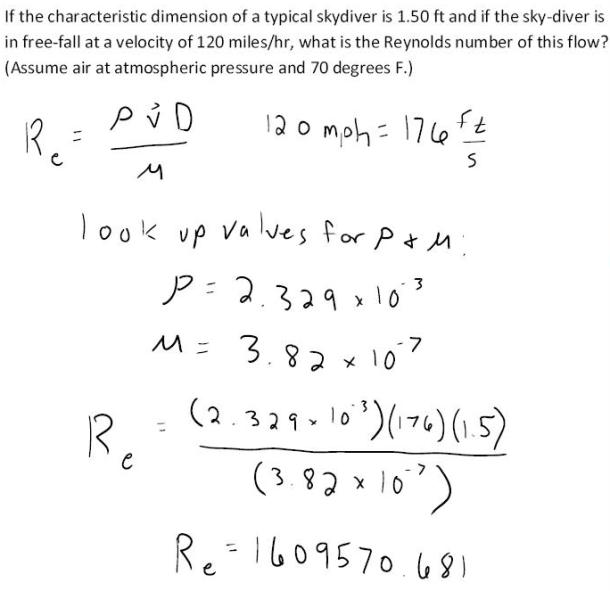fluid mechanics math problems engineering equations fluid mechanics basic mechanics school homework engineering math fluids formulas fluid mechanics problems fluid mechanics problem solutions to fluids problems full solution fluid mechanics engineering fluids problem solution fluid mechanics math problems engineering equations fluid mechanics basic mechanics school homework engineering math fluid mechanics formulas fluid problems fluid mechanics problem solutions to fluid mechanics problems full solution fluid mechanics engineering fluid mechanics problem solution
fluid mechanics math problems engineering equations fluid mechanics basic mechanics school homework engineering math fluids formulas fluid mechanics problems fluid mechanics problem solutions to fluids problems full solution fluid mechanics engineering fluids problem solution fluid mechanics math problems engineering equations fluid mechanics basic mechanics school homework engineering math fluid mechanics formulas fluid problems fluid mechanics problem solutions to fluid mechanics problems full solution fluid mechanics engineering fluid mechanics problem solution
Highalphabet Home Page fluid mechanics solutions fluids math solved Fluid Mechanics Page 1
If the characteristic dimension of a typical skydiver is 1.5 ft and if the sky-diver is in free-fall at a velocity of 120 miles/hr, what is the Reynolds number of this flow? (Assume air at atmospheric pressure and 70 degrees F.)If the characteristic dimension of a typical skydiver is 1.5 ft and if the sky-diver is in free-fall at a velocity of 120 miles/hr, what is the Reynolds number of this flow? (Assume air at atmospheric pressure and 70 degrees F.)